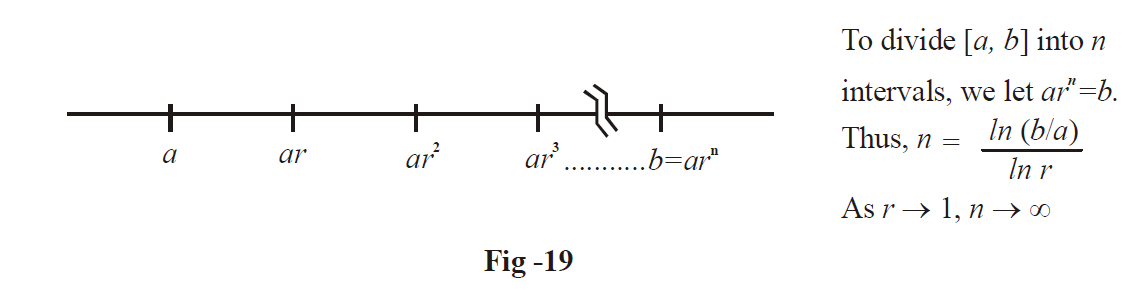# Definite Integrals as Limits of Sums

In this section, we will very briefly revisit the discussion we had in the unit “Integration Basics” on viewing integration as a limit of a sum. If you do not remember that discussion, you are advised to refer to it again.

In that discussion, we saw that the area under the curve is the sum of an infinitely large number of rectangles with infinitesimally small widths. These rectangles precisely give the area under the curve in the limit that their number tends to infinity, i.e.,:

\begin{align}&\int\limits_a^b {f(x)dx = \mathop {\lim }\limits_{n \to \infty } \sum\limits_{r = 1}^n {hf(a + rh)} } \\&where\quad h = \frac{{b - a}}{n}\end{align}

In particular, notice that if the lower limit a is 0, then

$\int\limits_0^b {f(x)dx = \mathop {\lim }\limits_{n \to \infty } \sum\limits_{r = 1}^n {\frac{b}{n}f\left( {\frac{{br}}{n}} \right)} } \,\,\,\,\,\,\,\,\,\,\,\,\,\,\,\,\,\,\,\,\,\,\,\,\,\,\,\,\,\,...(1)$

We sometimes encounter series of the form as in the right hand side of (1). The discussion above shows that such series can be summed using definite integrals.

This will become more clear through examples.

Example –24

(b) Find the sum of the series

\begin{align}&\frac{1}{n} + \frac{1}{{n + 1}} + \frac{1}{{n + 2}} + ...... + \frac{1}{{3n}}\text{as} \ n\to \infty \end{align}

(b) Find the sum of the series

\begin{align}&\frac{n}{{{n^2} + {1^2}}} + \frac{1}{{{n^2} + {2^2}}} + ...... + \frac{1}{{{n^2} + {n^2}}}\;\text{as}\;\ n\to \infty\end{align}

(c) Evaluate

$\mathop {\lim }\limits_{n \to \infty } \frac{{{{[(n + 1)(n + 2).....(n + n)]}^{1{\rm{/}}n}}}}{n}$

Solution: (a) The given series can be written concisely as

\begin{align}&S = \sum\limits_{r = 0}^{2n} {\frac{1}{{n + r}}} \\\,\,\,\,\, &\;\;= \frac{1}{n}\sum\limits_{r = 0}^{2n} {\frac{1}{{1 + (r{\rm{/}}n)}}} \end{align}

Comparing this with the right hand side of (1), we see that S can be expressed as the integral of a function \begin{align}f(x) = \frac{1}{{1 + x}}\end{align} from 0 to 2, because since r varies till 2n, $$r/n$$ varies till 2. Thus,

\begin{align}&S = \int\limits_0^2 {\frac{1}{{1 + x}}dx} \\\,\,\,\,\, &\;\;= \ln 3\end{align}

(b) Concisely put,

\begin{align}&S = \sum\limits_{l = 1}^n {\frac{n}{{{n^2} + {r^2}}}} \\\,\,\, &\;\;= \frac{1}{n}\sum\limits_{r = 1}^n {\frac{1}{{1 + {{(r{\rm{/}}n)}^2}}}} \end{align}

As discussed earlier, S can be written in integral form as

\begin{align}&S = \int\limits_0^1 {\frac{1}{{1 + {x^2}}}dx} \\\,\,\,\, &\;\;= \left. {{{\tan }^{ - 1}}x} \right|_0^1\\\,\,\,\, &\;\;= \frac{\pi }{4}\end{align}

(c) Let L represent the given limit. We have,

\begin{align}&\ln L = \frac{1}{n}\ln \left\{ {\left( {1 + \frac{1}{n}} \right)\left( {1 + \frac{2}{n}} \right)......\left( {1 + \frac{n}{n}} \right)} \right\}\\\,\,\,\,\,\,\,\,\,\, &\quad\;\;= \frac{1}{n}\sum\limits_{r = 1}^n {\ln \left( {1 + \frac{r}{n}} \right)} \end{align}

Thus, \begin{align}&\ln L = \int\limits_0^1 {\ln (1 + x)dx} \\\,\,\,\,\,\,\,\,\, &\quad\;\;= \left. {x\ln (1 + x)} \right|_0^1 - \int\limits_0^1 {\frac{x}{{1 + x}}dx} \\\,\,\,\,\,\,\,\,\, &\quad\;\;= \ln 2 - \int\limits_0^1 {\left( {\frac{{x + 1 - 1}}{{x + 1}}} \right)dx} \\\,\,\,\,\,\,\,\,\, &\quad\;\;= \ln 2 - 1 + \ln 2\\\,\,\,\,\,\,\,\,\, &\quad\;\;= 2\ln 2 - 1\\\,\,\,\,\,\,\,\, &\quad\;\;= \ln (4{\rm{/}}e)\end{align}

This implies that

$L = \frac{4}{e}$

From these three examples, the usefulness of definite integrals in summing series should be quite apparent.

One last thing about definite integration as the limit of a sum form: when we divide the area we want to evaluate into n rectangles, we need not have those n rectangles of the same width. The widths can be arbitrary as long as all of them tend to 0 in the limit  $$n\to \infty$$ . For example, we could divide the interval [a, b] as follows:In the scheme of division above, as $$r \to 1\,\, ,n \to \infty$$. This is also a valid division scheme since the width of the k th interval is $$a{r^k} - a{r^{k - 1}}$$ which tends to 0 as $$r \to 1\,\,{\rm{or }}\;n \to \infty$$ .

In fact, sometimes a uniform division scheme might not work due to the nature of the function to be integrated. In such cases, the division scheme described above could be used.

Example –25

Evaluate the integral $$\int\limits_a^b {\frac{1}{x}dx}$$ using first principles.

Solution: As described earlier, we divide the interval [a, b] into n non-uniform sub intervals, the k th interval being of width $${w_k} = a{r^k} - a{r^{k - 1}} = a{r^{k - 1}}(r - 1).$$ The sum of the areas of the n rectangles is

\begin{align}&S = \sum\limits_{k = 1}^n {{w_k}\,f(a{r^{k - 1}})} \\\,\,\,\,\, &\;\;= \sum\limits_{k = 1}^n {a{r^{k - 1}}(r - 1) \cdot \frac{1}{{a{r^{k - 1}}}}} \\\,\,\,\,\, &\;\;= \sum\limits_{k = 1}^n {(r - 1)} \\\,\,\,\,\, &\;\;= n(r - 1)\\\,\,\,\,\, &\;\;= \frac{{(r - 1)\ln (b{\rm{/}}a)}}{{\ln r}}\\\,\,\,\,\, \\&\;\;= \frac{{p\ln (b{\rm{/}}a)}}{{\ln (p + 1)}} & & & \left( \begin{array}{l}{\rm{where }}\;p = r - 1;\\{\rm{as }}r \to 1,p \to {\rm{0}}\end{array} \right)\end{align}

Thus, \begin{align}&\mathop {\lim }\limits_{n \to \infty } S = \mathop {\lim }\limits_{r \to 1} S = \mathop {\lim }\limits_{p \to 0} S = \ln (b{\rm{/}}a)\\\,\,\,\,\,\,\,\,\,\,\,\, &\quad\;\Rightarrow\quad \,\int\limits_a^b {\frac{1}{x}dx = \ln \left( {\frac{b}{a}} \right)} \end{align}

## TRY YOURSELF - II

Q. 1   Prove that  \begin{align}&\int\limits_0^\pi {\frac{{x\sin x}}{{2 - {{\sin }^2}x}}dx = \frac{{{\pi ^2}}}{4}}\end{align}

Q. 2   Prove that  \begin{align}&\int\limits_0^\pi {\frac{{{x^2}\cos x}}{{{{(1 + \sin x)}^2}}}dx = \pi (2 - \pi )}\end{align}

Q. 3   Prove that  \begin{align}&\int\limits_0^\pi {\ln (1 + \cos x)dx = - \pi \ln 2}\end{align}

Q. 4   Prove that  \begin{align}&\int\limits_0^\pi {\frac{x}{{1 + \sin \alpha \sin x}}dx = \frac{\pi }{{\cos \alpha }}\left( {\frac{\pi }{2} - \alpha } \right)}\end{align}

Q. 5   If y =  \begin{align}&\int\limits_0^{{x^2}} {\ln (1 + x)dx,\,\,\,{\rm{show that}}\,\,\frac{{dy}}{{dx}} = 2x\ln (1 + {x^2})}\end{align}

Q. 6   Prove that  \begin{align}&\int\limits_a^b {\left( {[x] + [ - x]} \right)dx = a - b}\end{align}

Q. 7   Show that  \begin{align}&\int\limits_0^{\pi {\rm{/}}2} {\sin 2x\ln (\tan x)dx = 0} \end{align}

Q. 8   Show that  \begin{align}&\int\limits_0^\pi {\frac{x}{{1 + {{\cos }^2}x}}dx = \frac{{{\pi ^2}}}{{2\sqrt 2 }}}\end{align}

Q. 9    If {I_n} =\begin{align} \int\limits_0^1 {{x^n}{{\tan }^{ - 1}}x\,\,dx,}\end{align} prove that (n + 1){I_n} + (n - 1){I_{n - 2}} =\begin{align} \frac{\pi }{2} - \frac{1}{n}\end{align}

Q. 10  Evaluate  \begin{align}&\mathop {\lim }\limits_{n \to \infty } \frac{{{{(n!)}^{1{\rm{/}}n}}}}{n}\end{align}